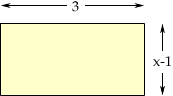Quandaries and Queries Who is asking: Other Level: Secondary Question: Hi - I have a measurement question that involves using the substitution method for algebra. The answer is 2.5 but I don't know how the answer was derived. The question is as follows: The rectange has an area of M square units and a perimeter of 2M units. What is the value of x? The length of the rectange is 3, while the width of the rectange is x-1. Thank you. Hi Sandy, I drrew a diagram of a rectangle and indicated the lengths of the sides.The area of a rectangle is the length times the width and hence the area of this rectangle is 3(x-1) = 3x - 3 But the area is M so 3x - 3 = M The perimeter of this rectangle is 3 + (x-1) + 3 + (x-1) = 6 + 2x -2 = 2x + 4 But the perimeter is 2M and hence 2x + 4 = 2M or x + 2 = M Hence M = 3x - 3 = x + 2 Solve for x. Penny Go to Math Central